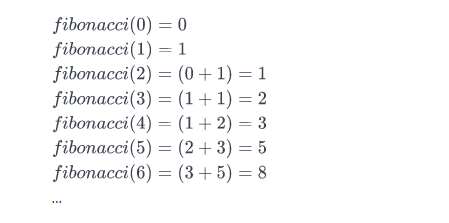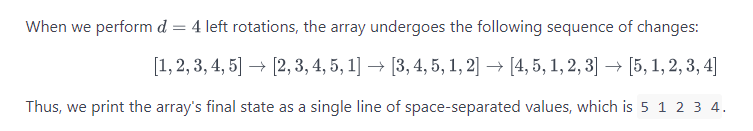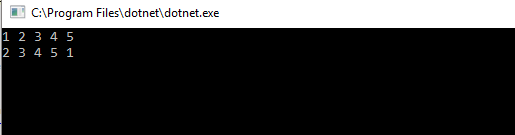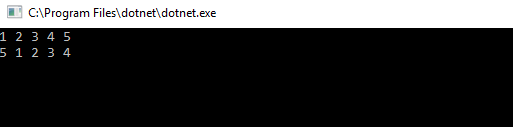## Find the Nth Fibonacci Number – C# Code

The Fibonacci sequence begins with Fibonacci(0) = 0  and Fibonacci(1)=1  as its respective first and second terms. After these first two elements, each subsequent element is equal to the sum of the previous two elements.

Here is the basic information you need to calculate Fibonacci(n):

• Fibonacci(0) = 0
• Fibonacci(1) = 1
• Fibonacci(n) = Fibonacci(n-1) + Fibonacci(n-2)

Given n, complete the fibonacci function so it returns Fibonacci(n).

Input Format

Nth number

Output Format

Fibonacci() Nth number.

Sample Input

Sample Output

Explanation

Consider the Fibonacci sequence:We want to know the value of Fibonacci(3). If we look at the sequence above,Fibonacci(3)  evaluates to 2. Thus, we print 2 as our answer.

## Array Left Rotation by D – C# Code

left rotation operation on an array of size n shifts each of the array’s elements 1 unit to the left. For example, if 2 left rotations are performed on array [1,2,3,4,5], then the array would become  [3,4,5,1,2].

Given an array of  n integers and a number, d , perform d left rotations on the array. Then print the updated array as a single line of space-separated integers.

Input Format

The first line contains two space-separated integers denoting the respective values of  (the number of integers) and  (the number of left rotations you must perform).
The second line contains  space-separated integers describing the respective elements of the array’s initial state.

Output Format

Print a single line of  space-separated integers denoting the final state of the array after performing  left rotations.

Sample Input

Sample Output

ExplanationCode

We can see the results for 1 and 4 rotations as follows: